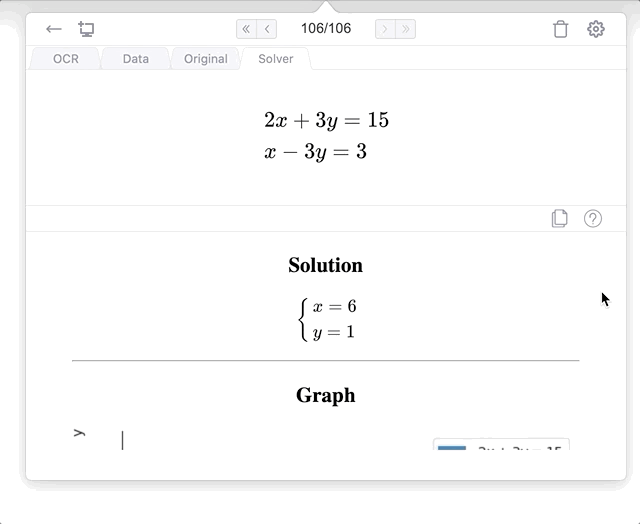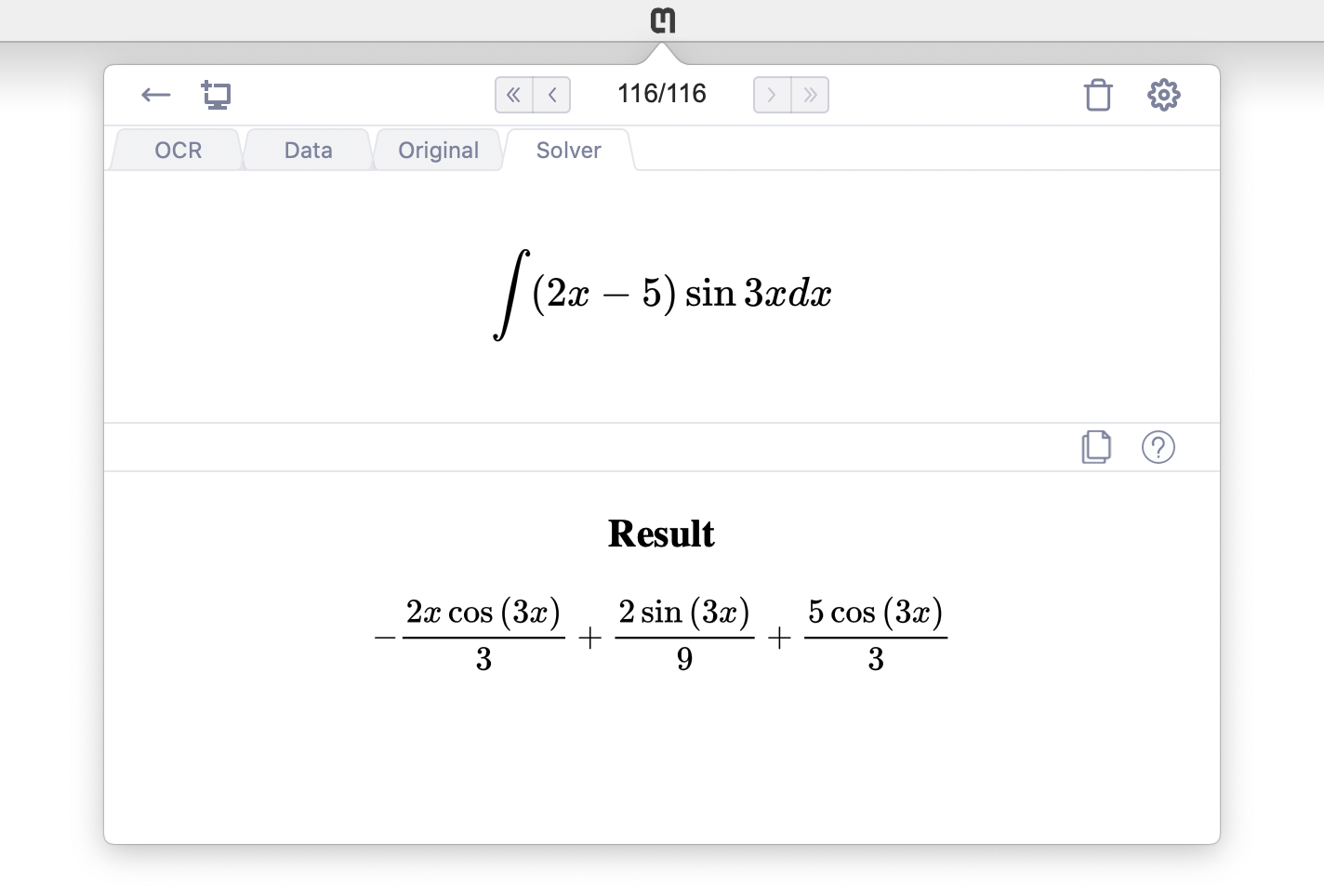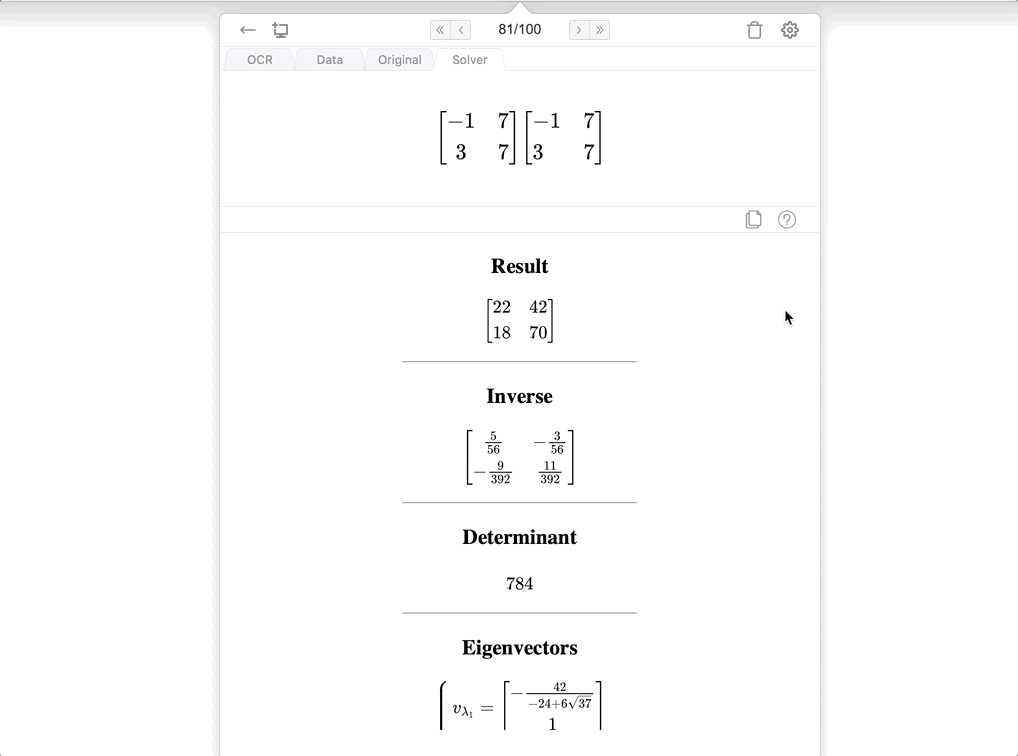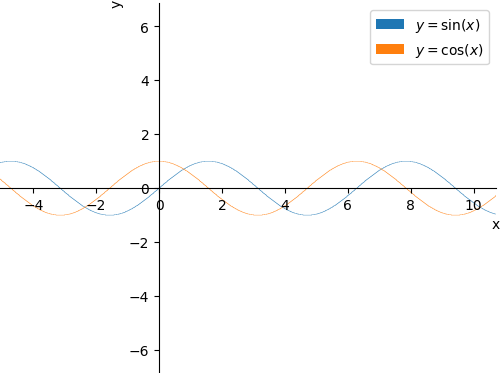# New Solver tab in Snip

By Kaitlin Cunningham

# Features

The Solver works especially well for:
• Calculating arithmetic
• Solving and graphing algebraic equations, systems of equations, and inequalities
• Computing integrals
• Performing matrix operations (will return eigenvector, eigenvalues, and more)
Here are some examples of what the Solver can do:

### Example 1: Graph system of equations

Snip systems of equations, get the solution and a graph:### Example 2: Compute integrals

Snip an integral and get the result:### Example 3: Matrix multiplication

Snip a matrix multiplication problem, get the solution, inverse, determinant, eigenvectors, eigenvalues, characteristic polynomial, dimensions, and multiplicities:# Sharing Solver information

The results of the Solver can easily be shared and pasted into any editor that supports Mathpix Markdown, like Snip Notes. Just click the copy button on the Solver tab to copy the results to your clipboard.
Here is what a result looks like when pasted into a Markdown document for this equation:
Solver result:
### Solution
$\left\{ \begin{array} {l} \,x = \frac{\pi}{4}\,,\quad y = \frac{\sqrt{2}}{2} \\\, x = \frac{5 \pi}{4}\,,\quad y = - \frac{\sqrt{2}}{2} \end{array} \right.$

---
### Graph
![Plot](https://cdn.mathpix.com/solve/images/f4a920358c7018e5bfc2e4b553b88305.png)


### Graph